# Law of Conservation of Mechanical Energy

This lecture, we’re finally going to do some calculations using energy!
I put a lot of energy into writing this article because of its importance, and it ended up being quite long.

## What is Mechanical energy?

Previously, we learned about kinetic energy and potential energy.
An object in motion has kinetic energy, while an object at a distance from a reference point has potential energy.

Then, for example, “an object moving at a high place” has both kinetic and potential energy.
In such a case, let’s treat kinetic energy and potential energy together!
This is the concept of mechanical energy.

“Together” means exactly what it says: just add them up.
Mechanical energy ＝ Kinetic energy ＋ Potential energy

In other words, if you are asked to find the mechanical energy, you can find the kinetic energy and potential energy using the formulas for each, and then add them together.

In mechanics, kinetic and potential energy are almost never used alone.
They are usually added together and treated in the form of mechanical energy.

## [Example] Free fall

The advantage of considering mechanical energy is the Law of Conservation of Mechanical Energy!

By the way, does mechanical energy really conserve?
Let us take free fall as an example.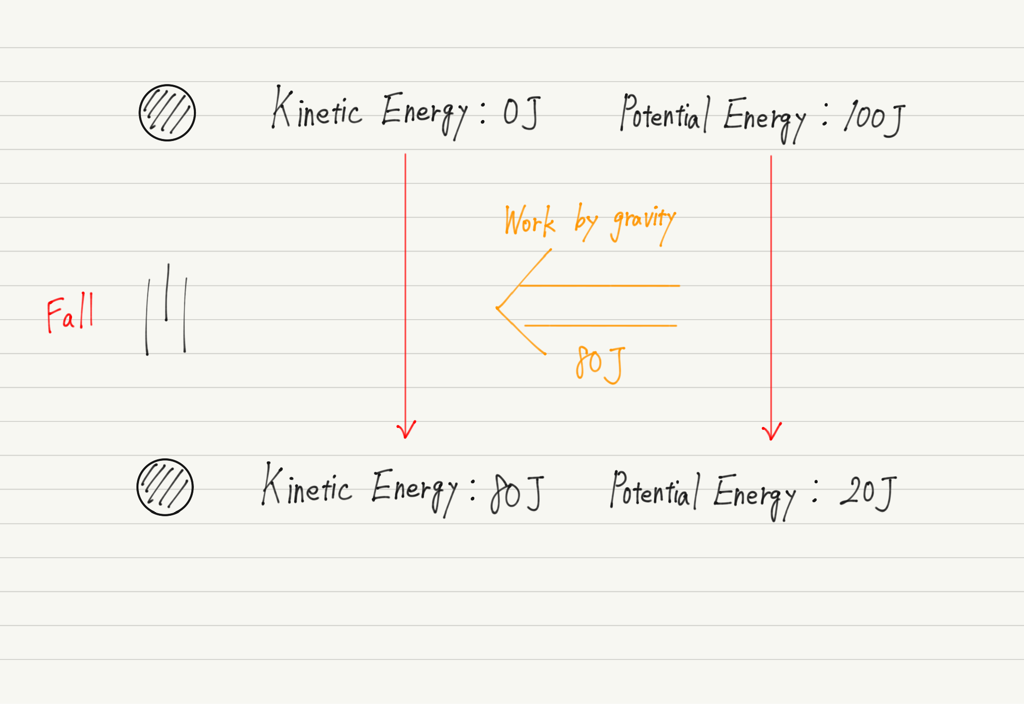First, an object is dropped from a point with a potential energy of 100 J (free fall has an initial velocity of 0, so the kinetic energy is also 0).

As an object falls, its height decreases, and therefore its potential energy also decreases.

Remember that “Energy = the ability to do work”.
If work is done, energy is reduced, and conversely, if work is done, energy is stored.

In the figure above, the potential energy has been reduced from 100J to 20J, but the reduced 80J was used for work.
Obviously, that 80J of work was done by gravitational force!

On the other hand, the object stores energy as work is done to it.
Since the initial velocity of the object was 0 and the velocity is increasing due to the fall, the energy is stored as kinetic energy.

That is, through the work done by gravitational force, the potential energy has been transformed into kinetic energy!

Let me try to express what I have just said in the form of an equation.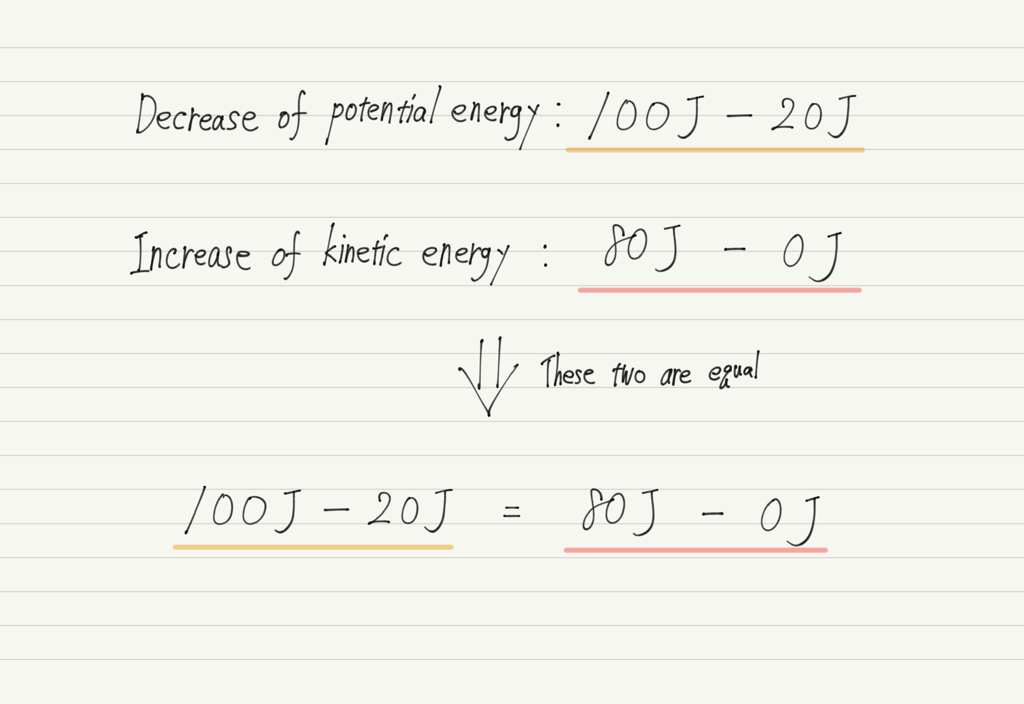Transpose negative terms.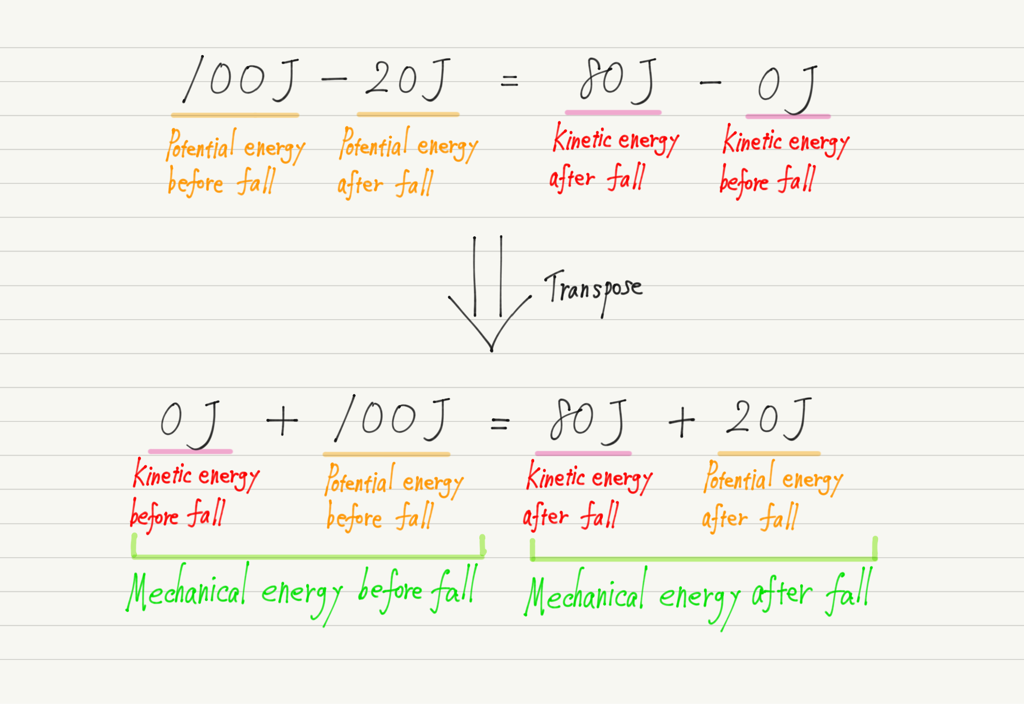What a surprise!
Both sides are in the form of “kinetic energy + potential energy”.
Here comes the kinetic energy!

## Strong points of the conservation law

If you look closely at the above equation, you will see that the “mechanical energy before the fall” and the “mechanical energy after the fall” are connected by an equal sign.

This equation asserts that as an object falls, its height and velocity change rapidly, but its mechanical energy remains the same.
This is the law of conservation of mechanical energy!

When something happens, we tend to focus on the change.
But this law tells us to focus not on what has changed, but on what has remained the same!

Is it that change is superficial and that the essence lies in what does not change?
Conservation laws will be found everywhere in the future, not just in mechanical energy.

## Notes on the Law

Forgive me for the rather lengthy preface, but it is an important law.
Here is a summary of the law of conservation of mechanical energy.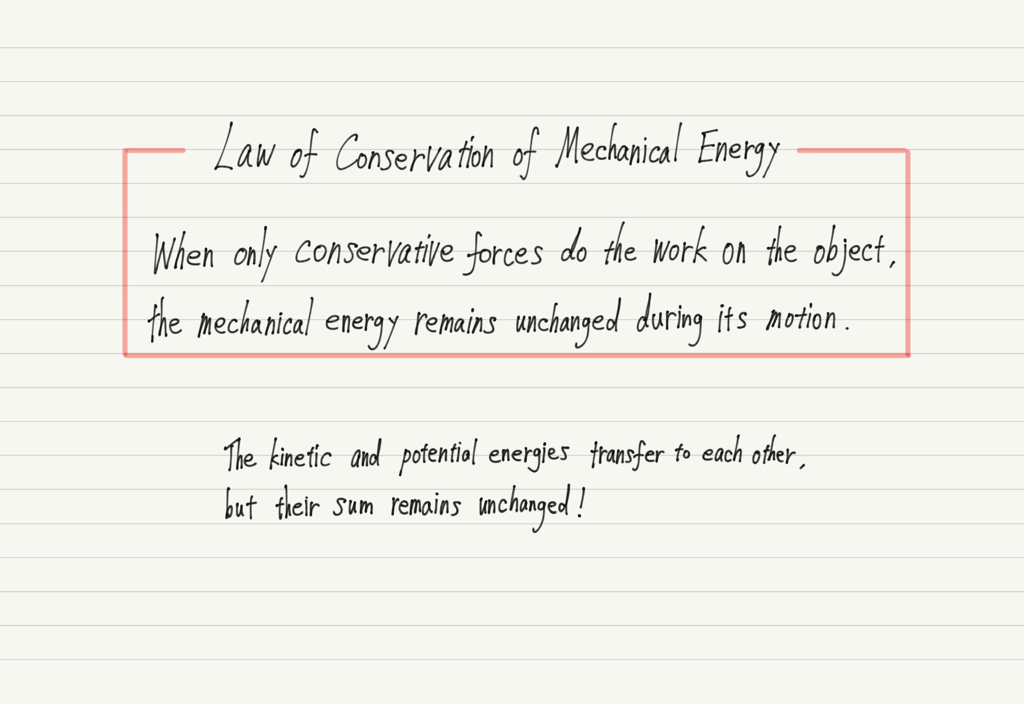First, let us discuss the situations in which this law is used.

The law of conservation of mechanical energy can be used “when there is a point in the motion for which the velocity and position are known (in many cases, the velocity and position of the starting point are given)”.

Once the velocity and position are known, the mechanical energy can be determined.
And since the mechanical energy does not change during motion, we can use this to obtain the velocity and position at another point.
We’ll calculate with examples later!

Before we do the examples, let me give you the biggest caveat in using the law of conservation of mechanical energy.

Mechanical energy is not unconditionally conserved!

Physical laws often take the form of “conditions → conclusion”.
The conclusion is important, but the condition must not be overlooked.

Again, if you look closely, you will see the condition “when only conservative forces do the work on the object”.

When nonconservative forces do work on an object, mechanical energy is unfortunately not conserved.
This is where the names conservative and nonconservative forces come from.
(Note that even if a nonconservative force is acting on an object, it will conserve if it is not doing any work.)

If there is friction or someone pushes an object with their hand, mechanical energy is no longer conserved.
Pay close attention to this point when solving the problem.

## Exercises

Solve the problems to understand how to use conservation laws.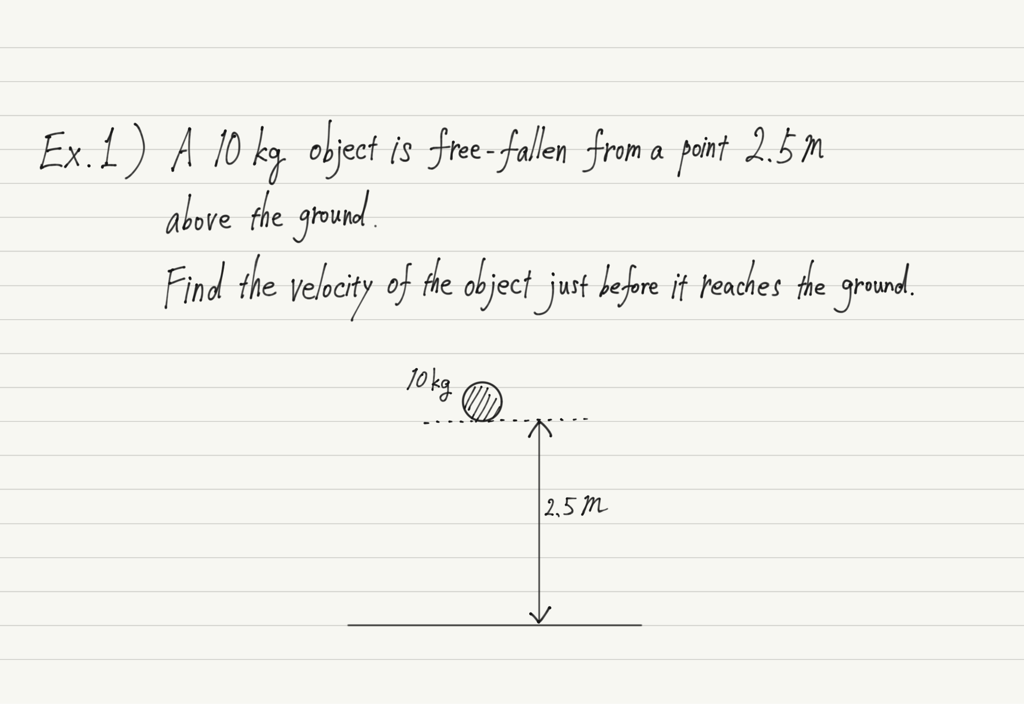This problem can be solved by using the formula for uniform accelerated motion.
But since we know the velocity just before free fall (0 m/s, of course) and the height from the ground, let’s solve it using the law of conservation of mechanical energy.

When using the law of conservation of mechanical energy, we first calculate the mechanical energy at the point where the velocity and position are known and at the point where the desired value exists.
In this case, the point where the velocity and position are known is before the fall, and the point where we want to find the value is just before the ground.

When determining energy, it is a good idea to use a table if you are not familiar with it.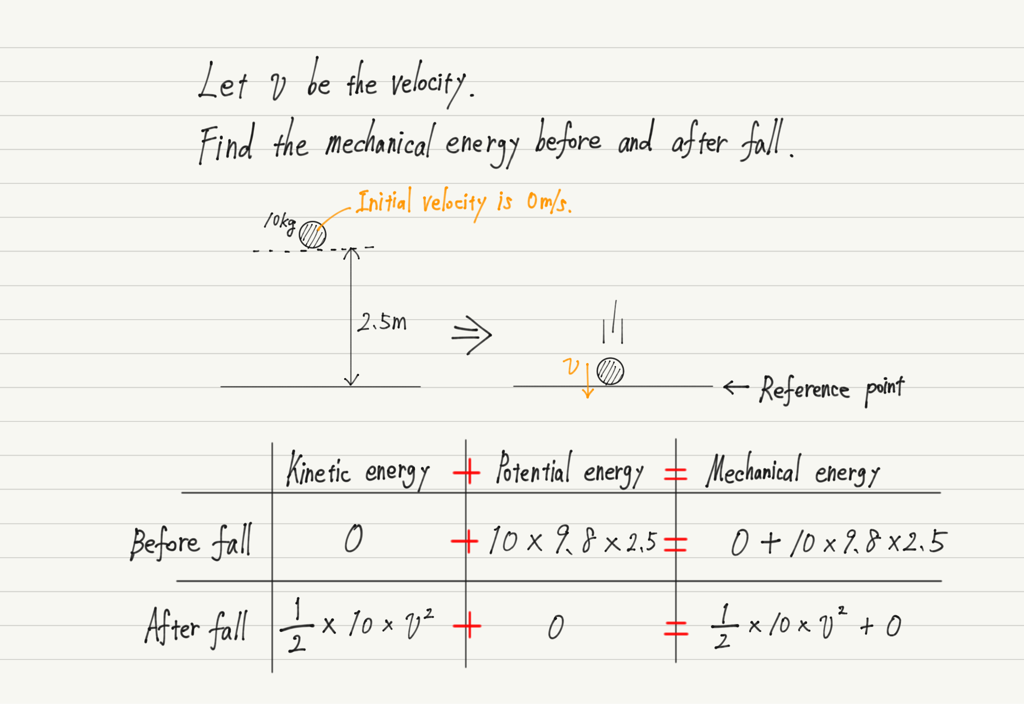First, we put the values we want to find in letters.
Then, using the formulas, we fill in the table by finding the potential energy and kinetic energy.

All that remains is to connect the mechanical energies by equality, but be sure to check the condition under which the law of conservation of mechanical energy can be used: “whether or not the nonconservative force is not doing any work”.

In this problem, the only force at work is gravitational force, so no problem!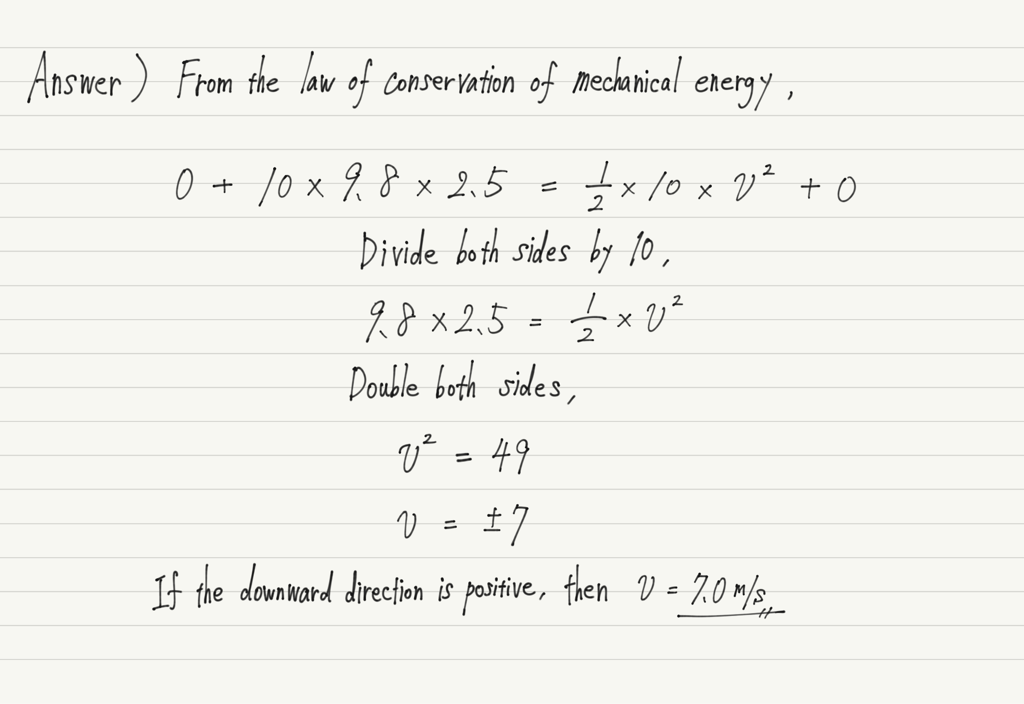The next problem involves elastic potential energy.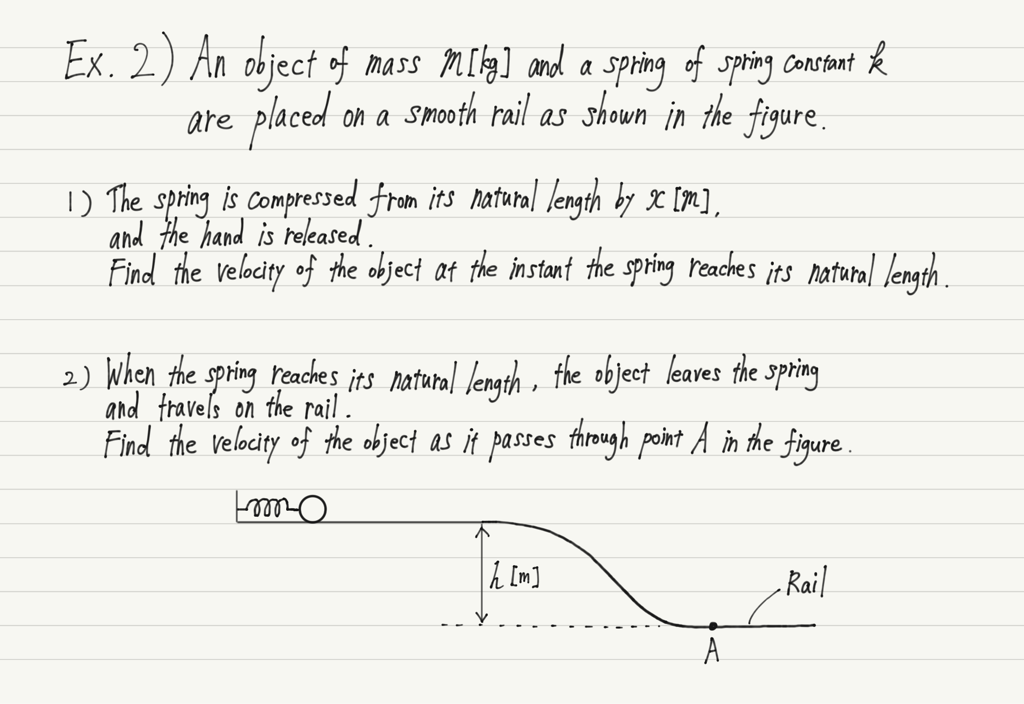First, check that nonconservative forces are not doing the work. The forces acting on the object are elastic force, gravitational force, and normal force from the rails.

Elastic force and gravitational force are conservative forces, so they are OK.
Normal force is a nonconservative force, but it does not do any work, so it is OK.
Thus, we can use the law of conservation of mechanical energy for this question, too!

The key to this problem is springs.
When springs are involved, the mechanical energy must be obtained by taking into account the elastic potential energy, but there is nothing special about springs.
Any potential energy can be added to kinetic energy to obtain mechanical energy.

The reference point for the potential energy is not specified in the problem, so you will have to determine it yourself.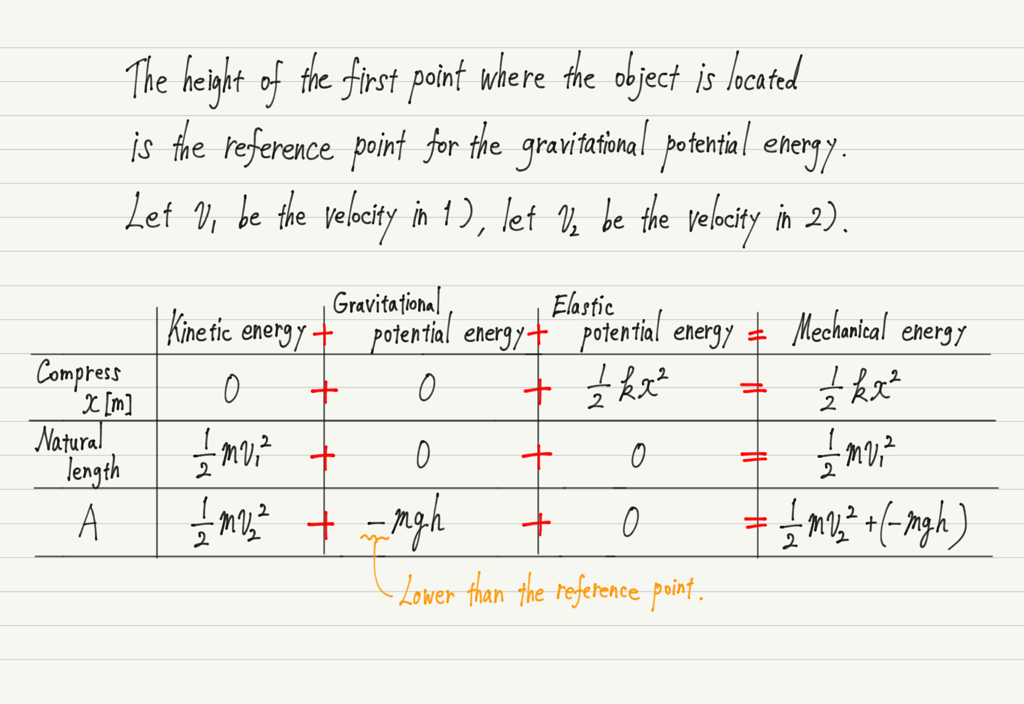There is one more column in the table because of the spring, but other than that, it is the same as before.
Now all we have to do is compare the mechanical energies!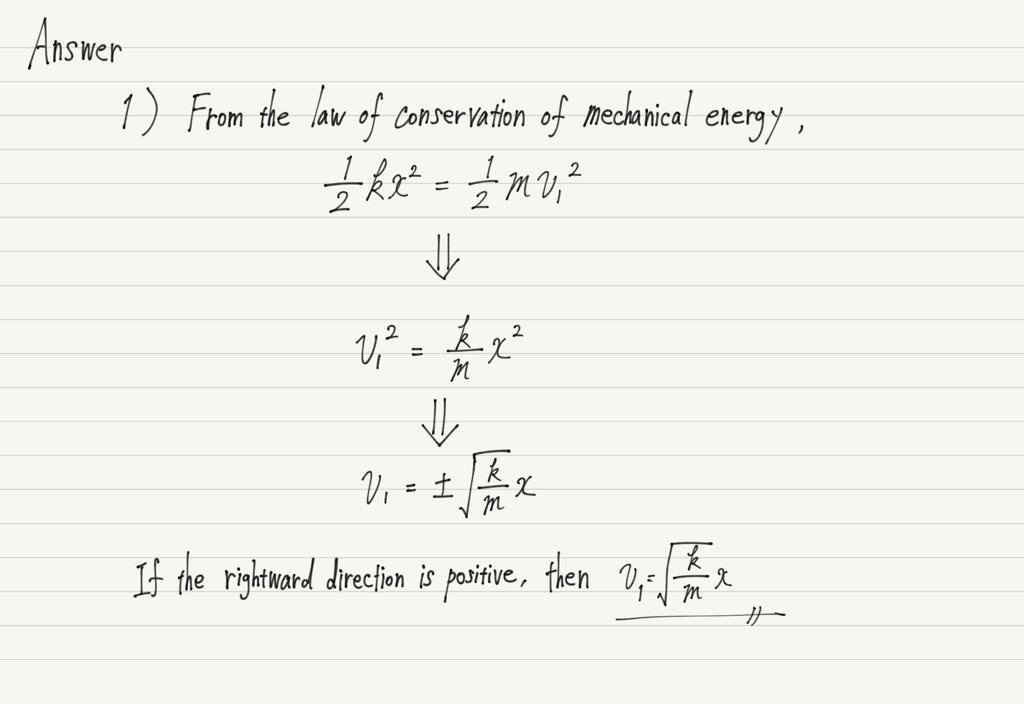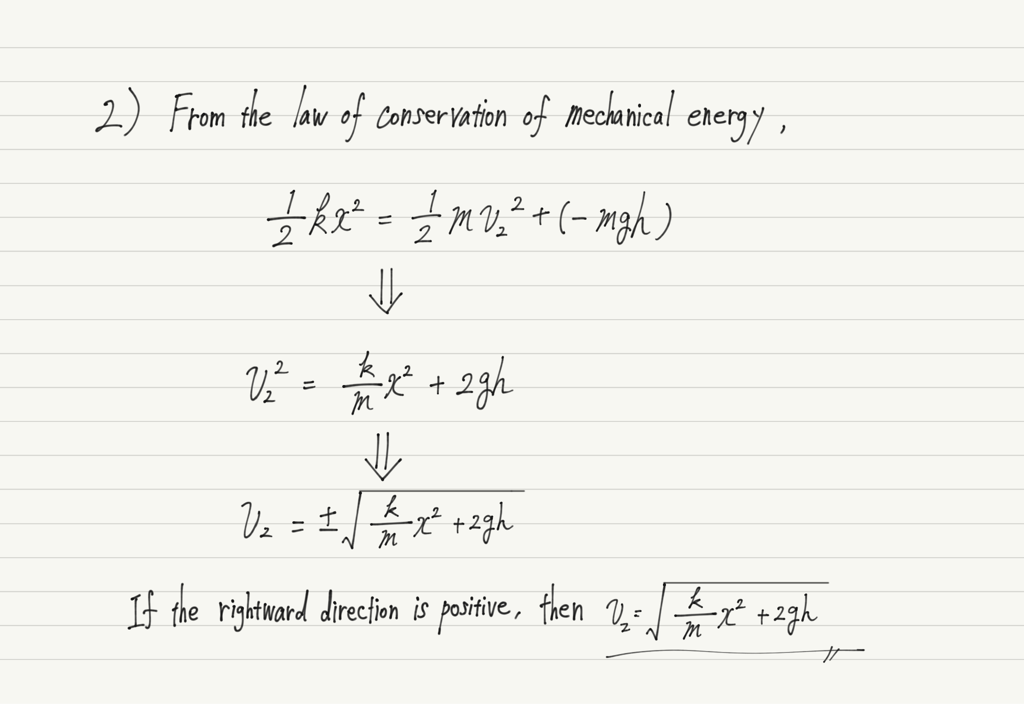This is how to solve problems using the law of conservation of mechanical energy.

The first thing you need to do is to remember the energy formula and be able to fill in the table by yourself.
If you do that, you should be able to solve the problem!

## Summary of this lecture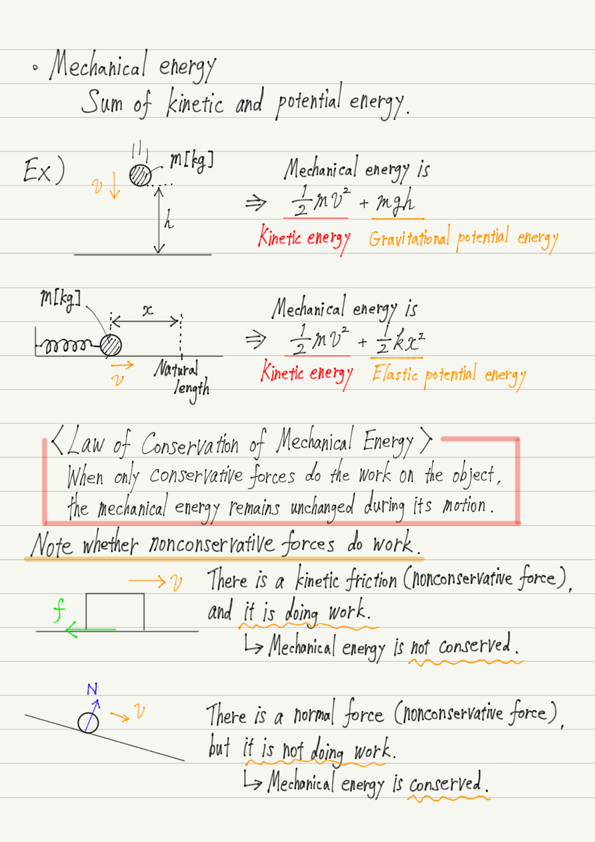## Next Time

In this lecture, we have noted that “when nonconservative forces do work, mechanical energy is not conserved.
If it is not conserved, of course the conservation law cannot be used to solve the problem.
Is there any other solution in such a case?

https://www.yukimura-physics.com/entry/dyn-f30

error: Content is protected !!